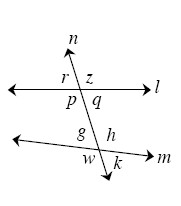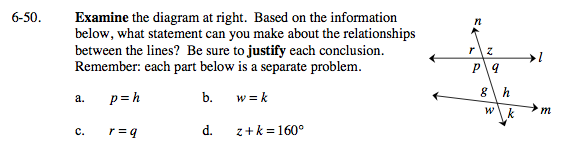Home > GC > Chapter 6 > Lesson 6.2.2 > Problem6-50

6-50.
1.the diagram at right. Based on the information below, what statement can you make about the relationships between the lines? Be sure to justify each conclusion. Remember: each part below is a separate problem. Homework Help ✎

1. p = h

2. w = k

3. r = q

4. z + k = 160°When do alternate interior angles have equal measures?

If p = h, then l and m are parallel.

If two angles which form a straight angle are equal, what must their measures be?

n is perpendicular to m because w and k are both 90°.

Under what circumstances are vertical angles equal?

Is there anything special about angles that add up to 160°?